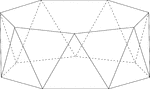Heptagonal/Septagonal Antiprism

Illustration of a heptagonal, or sometimes known as a septagonal antiprism. An antiprism is formed by…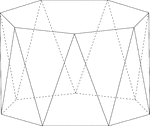Heptagonal/Septagonal Antiprism

Illustration of a heptagonal, or sometimes known as a septagonal antiprism. An antiprism is formed by…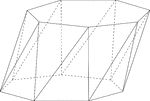Skewed Heptagonal/Septagonal Antiprism

Illustration of a skewed (non-right) heptagonal, or sometimes known as a septagonal antiprism. An antiprism…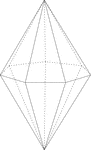Septagonal/Heptagonal Bipyramid

Illustration of a septagonal bipyramid. A bipyramid, or dipyramid, is formed by joining two congruent…Frustum Of A Decagonal Pyramid

Illustration of a hollow septagonal/heptagonal pyramid that has been cut by a plane parallel to the…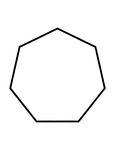Flashcard of a polygon with seven equal sides

A flashcard featuring an illustration of a polygon with seven equal sides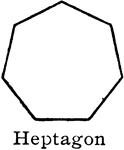Heptagon

"A polygon of...seven sides [is] a heptagon." —Hallock 1905Construction Of A Heptagon

An illustration showing how to construct a heptagon, or septagon. "The appotem a in a hexagon is the…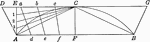Construction of Regular Heptagon in a Circle

Illustration of beginning of construction of a regular heptagon in a circle.Irregular heptagon

Illustration of an irregular polygon/heptagon/septagon.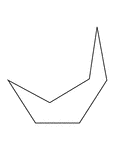Irregular Concave Heptagon

Illustration of an irregular concave heptagon.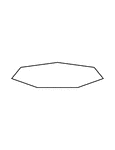Irregular Convex Heptagon

Illustration of an irregular convex heptagon. This polygon has some symmetry.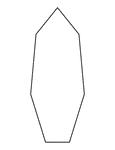Irregular Convex Heptagon

Illustration of an irregular convex heptagon. This polygon has some symmetry.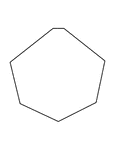Irregular Convex Heptagon

Illustration of an irregular convex heptagon. This polygon has some symmetry.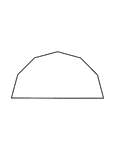Irregular Convex Heptagon

Illustration of an irregular convex heptagon. This polygon has some symmetry.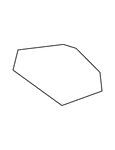Irregular Convex Heptagon

Illustration of an irregular convex heptagon.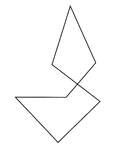Crossed Polygon

Illustration of crossed polygon. This is not generally considered to be a real polygon.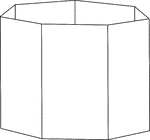Heptagonal/Septagonal Prism

Illustration of a hollow right heptagonal/septagonal prism with regular heptagons/septagons for bases…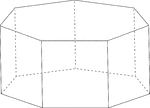Heptagonal/Septagonal Prism

Illustration of a regular right heptagonal/septagonal prism with regular heptagons/septagons for bases…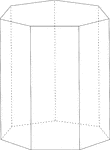Heptagonal/Septagonal Prism

Illustration of a right heptagonal/septagonal prism with regular heptagons/septagons for bases and rectangular…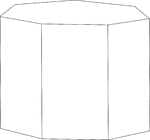Heptagonal/Septagonal Prism

Illustration of a right heptagonal/septagonal prism with regular heptagons/septagons for bases and rectangular…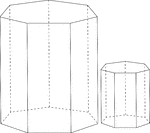Similar Heptagonal/Septagonal Prisms

Illustration of 2 similar right heptagonal/septagonal prisms. Both have regular heptagons/septagons…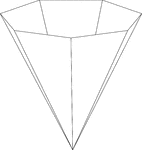Inverted Septagonal/Heptagonal Pyramid

Illustration of a hollow right heptagonal (septagonal) pyramid. The base is a heptagon and the faces…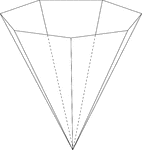Inverted Septagonal/Heptagonal Pyramid

Illustration of a hollow right heptagonal (septagonal) pyramid. The base is a heptagon and the faces…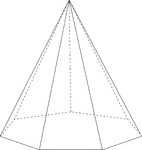Septagonal/Heptagonal Pyramid

Illustration of a right septagonal/heptagonal pyramid with hidden edges shown. The base is a heptagon…Septagonal/Heptagonal Pyramid Cut By Plane

Illustration of a right septagonal/heptagonal pyramid, with hidden edges shown, that has been cut by…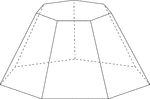Frustum Of A Septagonal/Heptagonal Pyramid

Illustration of a septagonal/heptagonal pyramid that has been cut by a plane parallel to the base. The…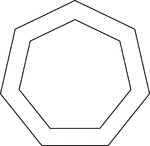2 Concentric Septagons/Heptagons

Illustration of 2 regular concentric heptagons/septagons.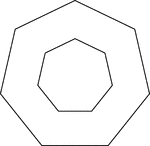2 Concentric Septagons/Heptagons

Illustration of 2 regular concentric heptagons/septagons.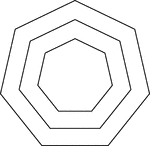3 Concentric Septagons/Heptagons

Illustration of 3 regular concentric heptagons/septagons that are equally spaced.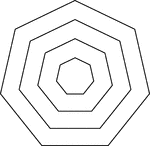4 Concentric Septagons/Heptagons

Illustration of 4 regular concentric heptagons/septagons that are equally spaced.